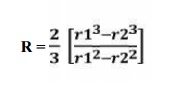Home | | Design of Transmission Systems | Design of a Disc or Plate Clutch

# Design of a Disc or Plate Clutch

Considering uniform pressure; When the pressure is uniformly distributed over the entire area of the friction face then the intensity of pressure.

DESIGN OF A DISC OR PLATE CLUTCH

Consider two friction surfaces maintained in contact by an axial thrust (W) .

Forces on a disc clutch.

Let    T = Torque transmitted by the clutch,

p = Intensity of axial pressure with which the contact surfaces are held

together,

r1 and r2 = External and internal radii of friction faces,

r = Mean radius of the friction face, and

μ = Coefficient of friction.

Consider an elementary ring of radius r and thickness dr

We know that area of the contact surface or friction surface = 2π r.dr

Normal or         axial force on the ring,

δW = Pressure × Area = p × 2 π r.dr

and the frictional force on the ring acting tangentially at radius r,

Frictional torque         Fr = μ × δW = μ.p × 2 π r.dr

acting on the ring,

Tr = Fr × r = μ.p × 2 π r.dr × r = 2 π μ p. r2.dr

We shall now consider the following two cases :

1. When there is a uniform pressure, and

2. When there is a uniform axial wear.

1. Considering uniform pressure. When the pressure is uniformly distributed over the entire

area of the friction face then the intensity of pressure,

p = W / π – [(r1)2 - (r2)2]

where W = Axial thrust with which the friction surfaces are held together.

We have discussed above that the frictional torque on the elementary ring of radius r and thickness dr is

Tr = 2π μ.p.r2.dr

Integrating this equation within the limits from r2 to r1 for the total friction torque. Total frictional torque acting on the friction surface or on the clutch,2. Considering uniform axial wear. The basic principle in designing machine parts that are subjected to wear due to sliding friction is that the normal wear is proportional to the work of friction. The work of friction is proportional to the product of normal pressure ( p) and the sliding velocity (V). Therefore,∝ ∝

Normal wear                        Work of friction      p.V

or p.V = K (a constant) or p = K/V ...(i)

It may be noted that when the friction surface is new, there is a uniform pressure distribution over the entire contact surface. This pressure will wear most rapidly where the sliding velocity is maximum and this will reduce the pressure between the friction surfaces.

This wearing-in process continues until the product p.V is constant over the entire surface. After this, the wear will be uniform.

Let p be the normal intensity of pressure at a distance r from the axis of the clutch. Since the intensity of pressure varies inversely with the distance, therefore

p.r = C (a constant) or p = C/r ...(ii)

and the normal force on the ring,

δW = p.2πr.dr

= (C/r) 2π r dr

2πC.dr

Total force acing=on the friction surface,

R = (r1 + r2)/2 = Mean radius of the friction surface.

Study Material, Lecturing Notes, Assignment, Reference, Wiki description explanation, brief detail
Mechanical : Design of Transmission Systems : Design of Cam Clutches and Brakes : Design of a Disc or Plate Clutch |

Related Topics